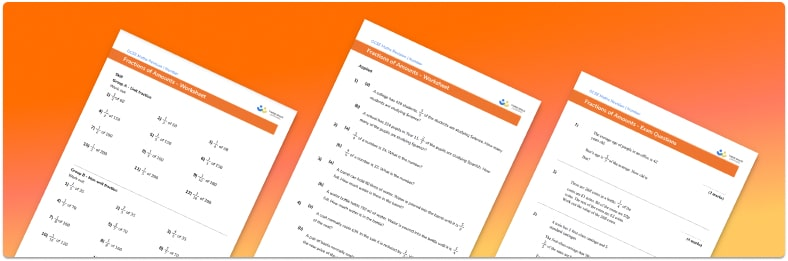# Fractions Of Amounts Worksheet• Section 1 of the fractions of amounts worksheet contains 36 skills-based fractions of amounts questions, in 3 groups to support differentiation
• Section 2 contains 4 applied fractions of amounts questions with a mix of word problems and deeper problem solving questions
• Section 3 contains 3 foundation and higher level GCSE exam style fractions of amounts questions
• Answer sheets and a mark scheme for all fractions of amounts questions are provided
• Questions follow variation theory with plenty of opportunities for students to work independently at their own level
• All maths resources created by fully qualified expert secondary maths teachers
• Suitable for GCSE maths revision for AQA, OCR and Edexcel exam boards

• This field is for validation purposes and should be left unchanged.

You can unsubscribe at any time (each email we send will contain an easy way to unsubscribe). To find out more about how we use your data, see our privacy policy.

### Fractions of amounts at a glance

Finding fractions of an amount is a key skill for proportional reasoning. The simplest case is considering unit fractions of amounts – for example, finding ⅕ of 10. It is important that students understand that finding one fifth is the same as dividing by the denominator 5.

Taking this a stage further, we consider non-unit fractions where the numerator is not 1, such as finding ⅗ of 10. Here we divide by 5 to find the value of ⅕ of the amount, then multiply by the numerator to find ⅗ as required. A bar model can be particularly useful when practising these basic ideas, so students can cut the bar into fifths and shade the appropriate amount.

When finding fractions of a number, the result may not always be a whole number and students may need to apply concepts from decimal arithmetic – for example, finding ¼ of 30. Students may also be asked to calculate fractions of quantities or measurements – for example, finding ¼ of 300g.

Looking forward, students can then progress to additional number worksheets, for example aor a.For more teaching and learning support on Number our GCSE maths lessons provide step by step support for all GCSE maths concepts.

## Do you have KS4 students who need more focused attention to succeed at GCSE?There will be students in your class who require individual attention to help them succeed in their maths GCSEs. In a class of 30, it’s not always easy to provide.

Help your students feel confident with exam-style questions and the strategies they’ll need to answer them correctly with our dedicated GCSE maths revision programme.

Lessons are selected to provide support where each student needs it most, and specially-trained GCSE maths tutors adapt the pitch and pace of each lesson. This ensures a personalised revision programme that raises grades and boosts confidence.

Find out more